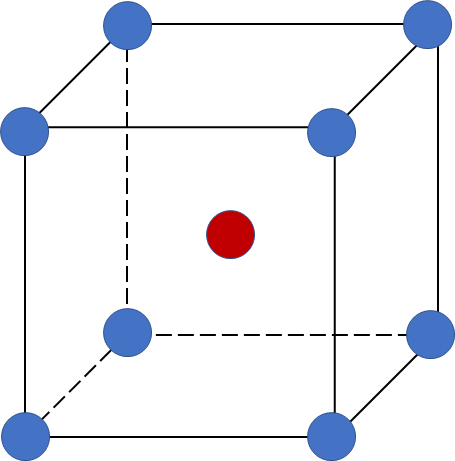# Problem: The CsCl structure is a simple cubic array of chloride ions with a cesium ion at the center of each cubic array (see Exercise 69). Given that the density of cesium chloride is 3.97 g/cm3, and assuming that the chloride and cesium ions touch along the body diagonal of the cubic unit cell, calculate the distance between the centers of adjacent Cs+ and Cl- ions in the solid. Compare this value with the expected distance based on the sizes of the ions. The ionic radius of Cs+ is 169 pm, and the ionic radius of Cl - is 181 pm.

###### FREE Expert Solution

A body-centered cubic (BCC) unit cell is composed of a cube with one atom at each of its corners and one atom at the center of the cube88% (448 ratings)###### Problem Details

The CsCl structure is a simple cubic array of chloride ions with a cesium ion at the center of each cubic array (see Exercise 69). Given that the density of cesium chloride is 3.97 g/cm3, and assuming that the chloride and cesium ions touch along the body diagonal of the cubic unit cell, calculate the distance between the centers of adjacent Cs+ and Cl- ions in the solid. Compare this value with the expected distance based on the sizes of the ions. The ionic radius of Cs+ is 169 pm, and the ionic radius of Cl - is 181 pm.

Frequently Asked Questions

What scientific concept do you need to know in order to solve this problem?

Our tutors have indicated that to solve this problem you will need to apply the Unit Cell concept. You can view video lessons to learn Unit Cell. Or if you need more Unit Cell practice, you can also practice Unit Cell practice problems.

What textbook is this problem found in?

Our data indicates that this problem or a close variation was asked in Chemistry: An Atoms First Approach - Zumdahl Atoms 1st 2nd Edition. You can also practice Chemistry: An Atoms First Approach - Zumdahl Atoms 1st 2nd Edition practice problems.